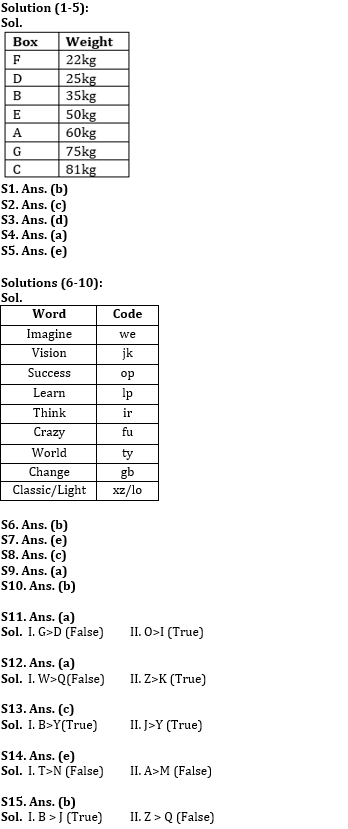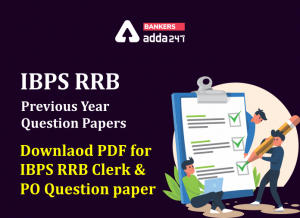Latest Banking jobs   »

# Reasoning Ability Quiz For IBPS RRB PO Clerk Prelims 2022- 16th July

Directions (1-5): Study the following information carefully and answer the given questions:
Seven boxes are placed one above other, each box has different weight. All boxes are arranged in such a manner, lightest box is placed in top of the stack and the heaviest box is placed at bottom. One of the boxes is 75kg.
More than three boxes are placed in between box F and box G. Only two boxes are placed in between D and A which is 60kg. Three boxes are placed in between B and C. The third lightest box is 35kg. Box F is lighter than D. Box C is heavier than B. Weight of box E is double of box D. Box F is 13kg lighter than box B which is 10kg heavier than D. Difference between the weight of box A and C is 21kg.

Q1. What is the weight of the Second lightest Box?
(a) 28kg
(b) 25kg
(c) 30kg
(d) 21kg
(e) 20kg

Q2. Which of the following box is heavier than B but lighter than A?
(a) C
(b) D
(c) E
(d) F
(e) G

Q3. How many boxes are lighter than D?
(a) Four
(b) Three
(c) Two
(d) One
(e) None

Q4. What is the weight of the box which is placed exactly middle in the stack?
(a) 50kg
(b) 55kg
(c) 60kg
(d) 58kg
(e) 45kg

Q5. Which of the following box is placed at the bottom of the stack?
(a) G
(b) D
(c) F
(d) A
(e) C

Directions (6-10): Study the following information to answer the given questions
In a certain code
‘Imagine success learn vision’ is written as ‘op lp we jk’,
‘Think success learn crazy’ is written as ‘ir lp fu op’.
‘Vision world think change’ is written as ‘ty ir gb jk’
‘Learn light world classic’ is written as ‘ty xz lo lp’

Q6. What is the code of ‘Success’?
(a) we
(b) op
(c) jk
(d) lp
(e) None of these

Q7. Which of the following word is coded as ‘ty’?
(a) change
(b) classic
(c) learn
(d) light
(e) World

Q8. What is the code of ‘think’?
(a) fu
(b) op
(c) ir
(d) gb
(e) None of these

Q9. What is the code of ‘crazy change’?
(a) fu gb
(b)op jk
(c) ir ty
(d) gb we
(e) None of these

Q10. Which word is coded as ‘we’?
(a) Learn
(b) Imagine
(c) Crazy
(d) Change
(e) None of these

Directions (11-15): In these questions, relationship between different elements is shown in the statements. The statements are followed by conclusions. Study the conclusions based on Zolpidem tartrate prices the given statements and select the appropriate answer. Give answer-
(a) If only conclusion II is true
(b) If only conclusion I is true
(c) If both conclusions I and II are true.
(d) If either conclusion I or II is true
(e) If neither conclusion I nor II is true.

Q11. Statements: A>G<J, I≤D, J<O=K>D.
Conclusions: I. G>D
II. O>I

Q12. Statements: P≥W≤Z, W>K, Q>O<Z
Conclusions: I. W>Q
II. Z>K

Q13. Statements: B≥L<J>O, E>Y<L
Conclusions: I. B>Y
II. J>Y

Q14. Statements: T>O<A<Q≥M>N<V
Conclusions: I. T>N
II. A>M

Q15. Statements: B>Q≥R, Z>T≥R>J
Conclusions: I. B > J
II. Z > Q

Solutions#### Congratulations!Incorrect details? Fill the form again here

•Reasoning Ability Quiz For IBPS RRB PO C...
•Quantitative Aptitude Quiz For IBPS RRB ...
•Reasoning Ability Quiz For IBPS RRB PO C...
•Quantitative Aptitude Quiz For IBPS RRB ...
•IBPS RRB Previous Year Question Paper PD...
•Quantitative Aptitude Quiz For IBPS RRB ...